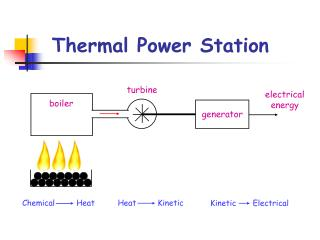DownloadDownload PresentationThermal Power Station

# Thermal Power Station

Download Presentation## Thermal Power Station

- - - - - - - - - - - - - - - - - - - - - - - - - - - E N D - - - - - - - - - - - - - - - - - - - - - - - - - - -
##### Presentation Transcript

1. turbine electrical energy boiler generator Heat Kinetic Chemical Heat Kinetic Electrical Thermal Power Station

2. turbines steam boiler generator electrical energy condenser

3. Reactor Turbine Generator Kinetic Electrical Heat Kinetic Nuclear Heat Nuclear Power Nuclear power stations operate similarly to thermal power stations, but instead of burning fossil fuels to produce heat, a nuclear reaction takes place inside a reactor.

4. Uranium is a fuel used in nuclear power stations. It is non-renewable, so it will eventually run-out. However, it has the advantage that a small amount of Uranium can produce huge amounts of energy. 1 kg of Coal 30 MJ 1 kg Uranium 5,000,000 MJ The major disadvantage of nuclear power is that the waste produced is radioactive. It has to be stored underground in lead and concrete containers for thousands of years.

5. Chain Reactions Neutrons Uranium Nucleus + ENERGY Neutron Fission Products A neutron is fired at a Uranium nucleus causing it to split-up into fragments. The splitting of the Uranium nucleus is called fission, so we call the fragments fission products. When the Uranium nucleus splits energy is released (which heats water in boiler). More neutrons are also released which can go on to split more Uranium nuclei. Control rods are used in nuclear reactors to absorb some of these new neutrons preventing too much energy being released in a short time.

6. Yellow Book Power Stations – Page 79 Q12, Q13, Q16, Q18, Q20, Q24

7. water flow low level reservoir turbine + generator Hydroelectric Power Station high level reservoir

8. transmission reservoir lines transformer generator water flow turbine

9. Water is stored at a height behind a dam. The water is allowed to flow down to a lower level. Potential Kinetic The water flows through a turbine spinning the blades which then turns the generator. Kinetic Electrical

10. Pumped Hydroelectric Power In a pumped hydroelectric power station water can be pumped back-up from the low level reservoir to the higher level. This usually happens throughout the night when the demand for electricity is lower. In the morning when we wake up and there is a demand for electricity, the water is allowed to flow back down again to generate the electricity we need.

11. ÷ x Potential Energy EP g m h Example 1 A dam stores 1.2 x 1010 kg of water and is 430 m above the turbine of a hydroelectric scheme. (a) Calculate the potential energy of the water in the dam. (b) The pipe delivers 1,280 kg of water to the turbine every second. Calculate the energy delivered to the power station in one second.

12. (a) (b) So there are 5.5 x 106 J of energy produced in one second. That means 5.5 x 106 J per second. But since The power output is 5.5 x 106 W, or 5.5 MW.

13. Question 1 A dam releases 750 kg of water down a pipe every second. The dam is 620 m above the turbine. (a) Calculate the potential energy of this amount of water at the start. (b) Calculate the power output of the power station if it were only 50% efficient. 4,650,000 J = 4.65 MJ 4.65 MJ = 4.65 MW 50% of 4.65 MW = 2.325 MW

14. Yellow Book Energy Conversions – Page 83 Q48 and Q51

15. Efficiency When an energy transfer takes place some energy is alwayswasted or degraded through heat. For example, in a lamp Electrical Light + Heat “Energy in” is the electrical energy. “Energy out” is the light energy. “Wasted energy” is the heat energy. If an energy transfer is efficient then only a small amount of energy is wasted. Most of the “energy in” is changed to useful “energy out”.

16. ÷ x Eout Ein Eff Some energy is always wasted so the efficiency is always less than 1. Sometimes the efficiency is written as a percentage, for example 1 can be written as 100% 0.75 can be written as 75% 0.37 can be written as 37% ( decimal is multiplied by 100 to give percentage )

17. Example 1 A nuclear power station produces 1,500 MJ of electrical energy. This requires 5,000 MJ of heat energy from the reactor. Calculate the efficiency of the power station. ** NO UNIT **

18. ÷ x Efficiency can also be calculated from input and output powers. Pout Pin Eff A thermal power station has a percentage efficiency of 33%. It produces 600 MW of electrical power. How much power needs to be produced in the boiler?

19. Yellow Book Efficiency – Page 80 Q25 (a), (d) and (f) Q28, Q30, Q31, Q33, Q34, Q36